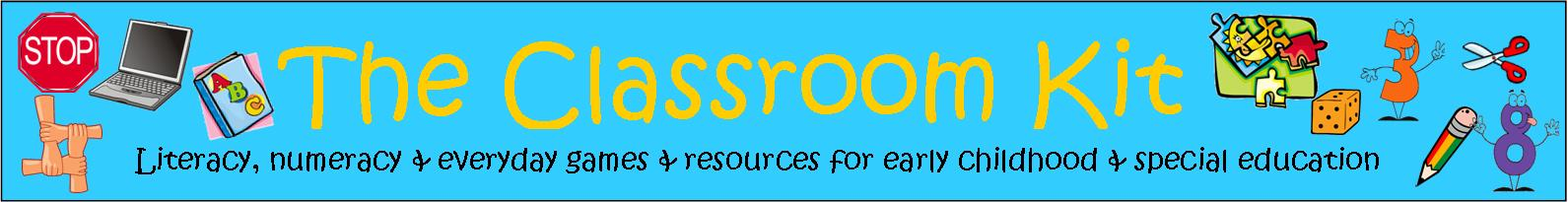## Maths Activities With Addition, Subtraction, Multiplication and DivisionIncorporating games into maths activities that focus on computations can be a productive teaching strategy. Traditionally, classroom experiences with computations have involved text-books or worksheets with rows of sums for students to complete. With card and board games, mental strategies can be developed, and automatic recall of computation facts is supported through enjoyable activities that are highly motivating. Early concepts include basic facts such as 1 more and 1 less. There are many games incorporating learning the friends of 10 and simple addition facts, and later on, concepts can include learning tables facts, percentages and decimals.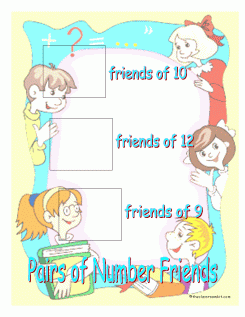Pairs of Number Friends (9,10,12) Game

A game with a base board and set of cards, where players use mental maths strategies to work out whether the total of each sum is 10 or 12 or 15. The format keeps players interested, as they quickly gain sets of cards. Having automatic recall of these simple number facts is central to being able to develop the ability to think mathematically.(missing pages added on 8th April 2015)

A game with a base board and set of cards, where players use mental maths strategies to work out whether the total of each sum is 10 or 12 or 15. The format keeps players interested, as they quickly gain sets of cards. A great addition to the class set of maths activities.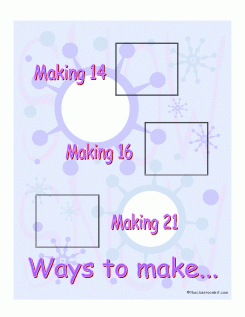A game with a base board and set of cards, where players use mental maths strategies to work out whether the total of each sum is 14, 16 or 21. Cards include addition, subtraction and multiplication facts. The format keeps players interested, as they quickly gain sets of cards. A great addition to the class set of maths activities.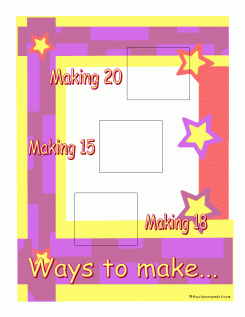Ways to Make -15 18 20- Game

A game with a base board and set of cards, where players use mental maths strategies to work out whether the total of each sum is 10 or 12 or 15. The format keeps players interested, as they quickly gain sets of cards. Having automatic recall of these simple number facts is central to being able to develop the ability to think mathematically.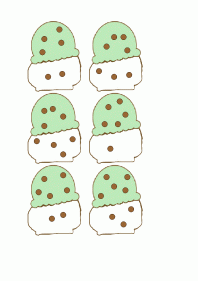A set of 24 cones and icecream scoops to combine. This activity aids in demonstrating how to combine numbers to find a total. It introduces the written algorithm for addition: 7+3=10Busy Bears Sums Under 10

A card game where players take turns to pull cards from the container and state aloud what the sum and the answer is. If it is correct, the player keeps it. The player with the most sum cards at the end of the game is the winner. This game provides lots of repetition with the automatic number facts students are learning. There are special cards to add interest to the game.Dragons and Knights: sums under 10

A card game where players take turns to pull cards from the container and state aloud what the sum and the answer is. If it is correct, the player keeps it. The player with the most sum cards at the end of the game is the winner. This game provides lots of repetition with the automatic number facts students are learning. There are special cards to add interest to the game.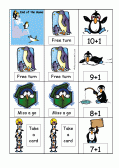A card game where players take turns to pull cards from the container and state aloud what the sum and the answer is. If it is correct, the player keeps it. The player with the most sum cards at the end of the game is the winner. This game provides lots of repetition with the automatic number facts students are learning. There are special cards to add interest to the game.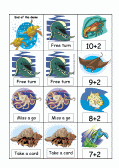Under The Ocean Card Game Free resource

A game to provide practise in mentally adding and subtracting 2 from small numbers.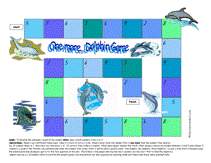A game to develop automatic number facts - 'one more than' numbers from 2 to 9.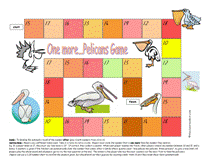A game to develop automatic number facts - 'one more than' numbers from 10 to 19.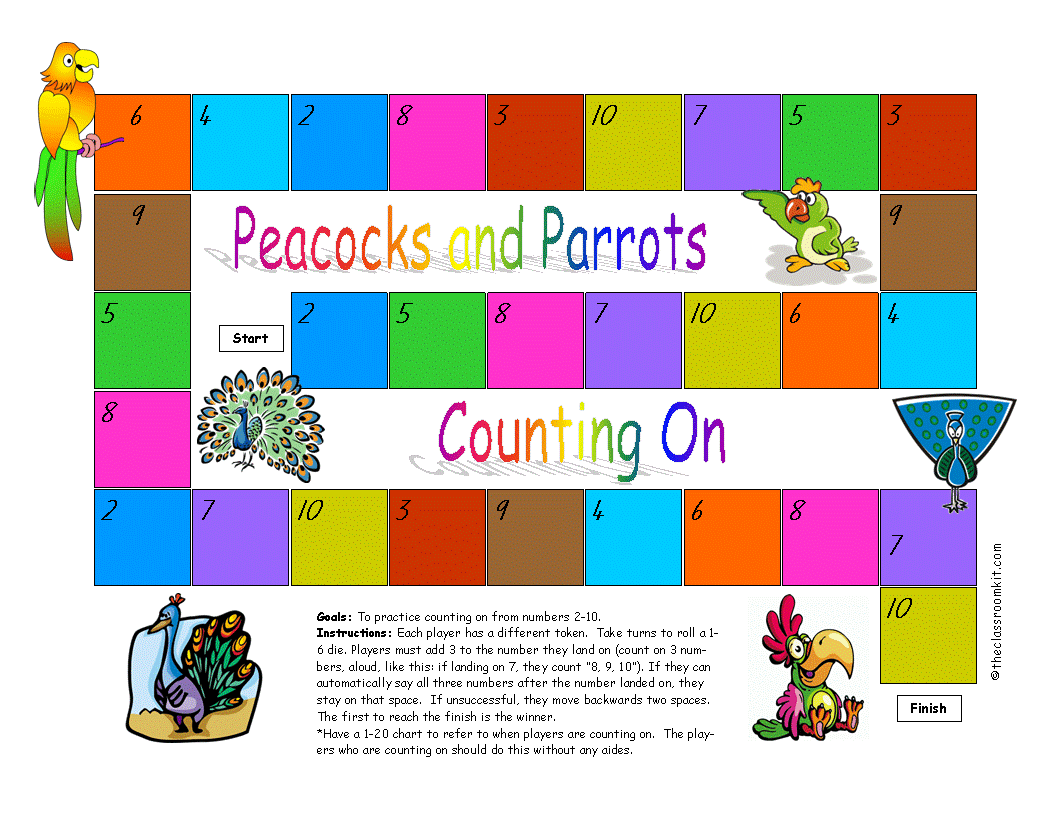A game to develop the skill of counting on 3 with numbers 2-9.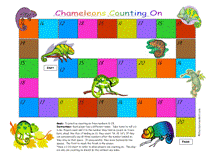Chameleons Counting On

A game to develop the skill of counting on 3 with numbers 11-20.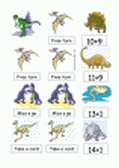Dinosaur Cards: Teen Sums

A card game where players take turns to pull cards from the container and state aloud what the sum and the answer is. If it is correct, the player keeps it. The player with the most sum cards at the end of the game is the winner. This game provides lots of repetition with the automatic number facts students are learning. There are special cards to add interest to the game.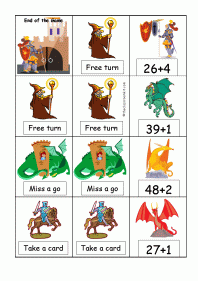Dragons and Knights (2-digit numbers simple sums)

A card game where players take turns to pull cards from the container and state aloud what the sum and the answer is. If it is correct, the player keeps it. The player with the most sum cards at the end of the game is the winner. The sums in this game encourage mental counting on and counting back, with the 2-digit number always presented first, and a single digit number next. Both addition and subtraction of small numbers are included.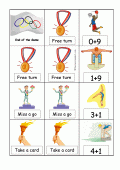Olympic Sums Under 10

Olympic Teen Sums

A card game where players take turns to pull cards from the container and state aloud what the sum and the answer is. If it is correct, the player keeps it. The player with the most sum cards at the end of the game is the winner. This game provides lots of repetition with the automatic number facts students are learning. There are special cards to add interest to the game.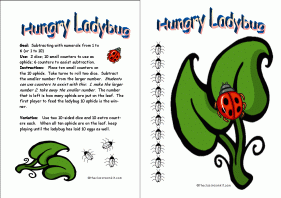The Hungry Ladybug Game Free resource

A board game that incorporates the concept of subtraction with numbers from 1 to 6 or 1 to 10.

## Developing efficient mental maths strategies: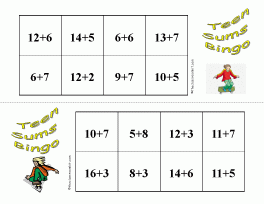Teen Sums Bingo Game

This game is great for developing efficient strategies for adding numbers up to 20. Players need to choose the best strategy for them, in order to calculate the totals quickly.Bridging Tens Bingo Game

Use this game to assist students who are developing the mental maths strategy of bridging tens.
Consists of 4 bingo boards, 4 sets of double tens frames and 24 sum cards.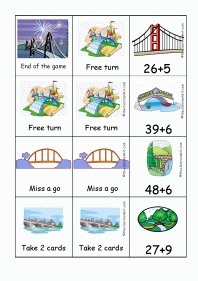Bridging Ten Card Game

Use this game to assist students who are developing the mental maths strategy of bridging tens.
Consists of 64 sum cards and 10 special cards.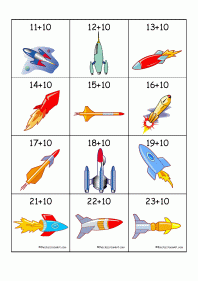Use this game to assist students who are developing mental maths strategies by adding ten to 2-digit numbers.
Consists of 72 sum cards and 10 special cards.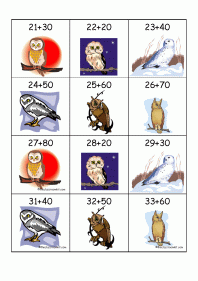Wise Old Owl - adding multiples of ten

Use this game to assist students who are developing mental maths strategies by adding multiples of ten (+20, +30, +40, +50, +60, +70, +80).
Consists of 60 sum cards and 10 special cards.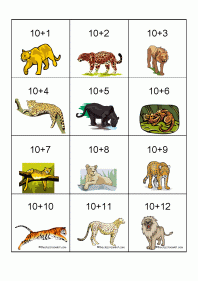Big Cats - Adding 10s Card Game

Use this game to assist students who are developing mental maths strategies by counting withs tens.
Consists of 51 sum cards and 9 special cards.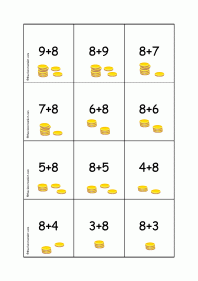Bridging Tens - Piggy Bank Game

Use this game to assist students who are developing the mental maths strategy of bridging tens. The sums included are ones where it is useful to bridge the tens.
Consists of 48 sum cards and 12 special cards.Magic Doubles Card Game

A card game where players take turns to pull cards from the container and state aloud what the sum and the answer is. If it is correct, the player keeps it. The player with the most sum cards at the end of the game is the winner. This game provides lots of repetition with the automatic number facts students are learning. There are special cards to add interest to the game.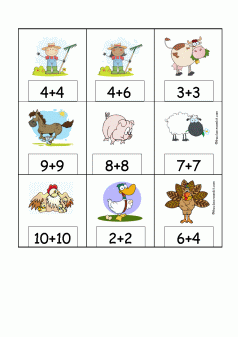Farmyard Friends of Ten and Doubles Card Game

A card game where players take turns to pull cards from the container and state aloud what the sum and the answer is. If it is correct, the player keeps it. The player with the most cards at the end of the game is the winner. This game provides lots of repetition with the automatic number facts students are learning. There are special cards to add interest to the game.Halloween Friends of 10 and Doubles Cards

A card game where players take turns to pull cards from the container and state aloud what the sum and the answer is. If it is correct, the player keeps it. The player with the most cards at the end of the game is the winner. This game provides lots of repetition with the automatic number facts students are learning. There are special cards to add interest to the game.Fairy Friends of 10 Board
A game to assist students in learning the 'friends of 10' combinations (8+2 9+1 6+4 5+5 3+7 10+0).

Take turns to roll two dice and state the total rolled. Each time you roll a friend of 10 pair, place a counter on one of the mushrooms. The first player to trap the fairy inside the 5 mushrooms gets their wish.Footy Friends of 10 Board A game to assist students in learning the 'friends of 10' combinations (8+2 9+1 6+4 5+5 3+7 10+0).

Take turns to roll two dice and state the total rolled. Each time you roll a friend of 10 pair, place a counter on one of the footballs. The first player to kick 5 goals wins.Pizza Friends of 10 Board A game to assist students in learning the 'friends of 10' combinations (8+2 9+1 6+4 5+5 3+7 10+0).

Take turns to roll two dice and state the total rolled. Each time you roll a friend of 10 pair, place a counter on one of the pizzas. The first player to take 5 pizza slices is the winner.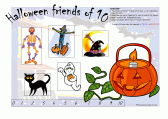Halloween Friends of Ten Board Free resource

A game to assist students in learning the 'friends of 10' combinations (8+2 9+1 6+4 5+5 3+7 10+0).

Played in the same way as Jungle Friends of Ten. Different boards can be used by each player during the same game, depending upon what interests each child.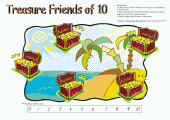Treasure Friends of 10 Board A game to assist students in learning the 'friends of 10' combinations (8+2 9+1 6+4 5+5 3+7 10+0).

Take turns to roll two dice and state the total rolled. Each time you roll a friend of 10 pair, place a counter on one of the treasure chests. The first player to collect 5 treasure chests is the winner.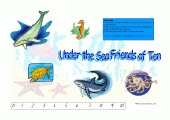Under The Sea Friends of 10 Board A game to assist students in learning the 'friends of 10' combinations (8+2 9+1 6+4 5+5 3+7 10+0).

Take turns to roll two dice and state the total rolled. Each time you roll a friend of 10 pair, place a counter on one of the sea creatures. The first player to spot 5 sea creatures is the winner.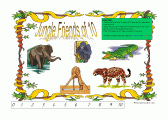Jungle Friends of Ten Board Free resource

A game to assist students in learning the 'friends of 10' combinations (8+2 9+1 6+4 5+5 3+7 10+0).

Take turns to roll two dice and state the total rolled. Each time you roll a friend of 10 pair, place a counter on one of the jungle animal. The first player to find 5 animals is the winner.

## Multiplication-Division Games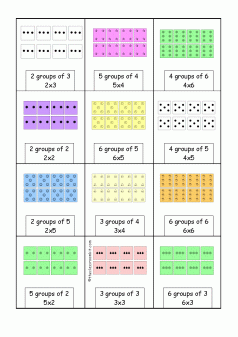Chocolate Counting Groups - card game (multiplication groups)

A card game where players have arrays to help them calculate the total. The computation is shown in both terms: 4 groups of 2 and 4x2, with multiple sets of 2 available to assist with the calculation. The player needs to know that they only need to count 4 of the groups to get the total. Players must check each others' calculations are accurate throughout the game. There is an answer card available to assist learners who are checking others' calculations if needed.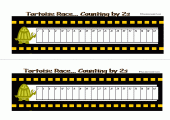Tortoise Race (counting by 2s and simple doubles)

(Best printed in A3 size)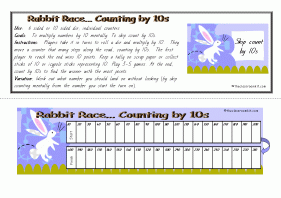Rabbit Race Free resource

(counting by 10s and multiplying by 10s)

(Best printed in A3 size)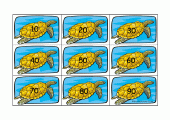Turtle Tens Game
Goal: To practise adding single digits to numbers that end in zero, and skip counting by tens.
Use: A deck of playing cards 1-10 with picture cards removed and turtle tens cards
Instructions: Shuffle both sets of cards and place in separate stacks. Deal one of each card to all players. Players add the numbers together mentally and compare their numbers. The player with the largest number scores 10 points. The winner is the first person to reach 100 points.## External Links to Online Games and Activities

Dartboard Game

Little Animals Activity Centre

Can You Balance?

National Library of Virtual Manipulatives (maths activities) (pre-k to Yr 12) Number operations, algebra, geomety, measurement and data analysis and probability

Post Sorting - calculations Can be used as a class game on an electronic whiteboard or individually. Tasks are timed and there are a range of skills covered.

Fractions- finding equivalent fractions resource http://www.crickweb.co.uk/ks2numeracy-tools.html#